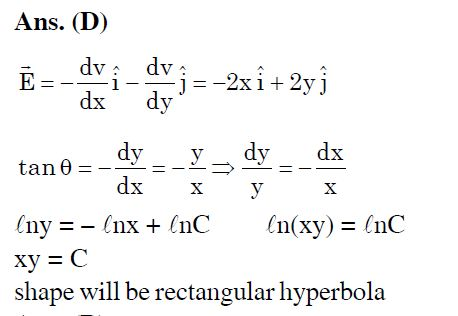# Doubt from electrostatics

2 Likes

from your solution we can point that D is most appropriate chose but
from this how would you know that electric field lines are reaching infinity at x=0 or y=0

For example :at x=0 E=2yj for y>0 we are sure that E is positive but now y can take any value from 0 TO infinity .now , how to draw field lines? how would we know that field lines will reach infinity only in above condition?

this is the solution.i am unable to understand why and how they found value of tan or dy/dx i.e how did they differentiate?We know that , \vec E=(\frac{-dv}{dx})\hat i +(\frac{-dv}{dy})\hat j and as per the question we got
\vec E=-2(x\hat i-y\hat j)
For the \vec E ,Tan{\theta} =\frac{(2y)}{(-2x)}=\frac{-y}{x}
,i.e \frac{dy}{dx}=Tan{\theta} (slope of the curve)
Now,
\rightarrow\frac{dy}{dx}=\frac{-y}{x},i.e
\rightarrow\frac{-dy}{y}=\frac{dx}{x}
\rightarrow-lny=lnx+lnc
\rightarrow ln(xy)=ln(c)
Therefore ,
\bbox[5px,border:2px solid red]{XY=C} is our desired curve equation,i.e Hyperbola